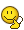# Trouble with another Kinematics question

## Homework Statement

A person riding a bike travels at an average velocity of 3m/s for 30min. The person then takes a water break for 15 min. The person finally travels a distance of 450m at an average velocity of 1.5m/s. What is the persons average velocity?

2. Homework Equations
[/B]
• Average Velocity: Δx/Δt
(Change in positions vs change in time)

• Conversion

3. The Attempt at a Solution

1.
I first labeled the data.

Trip1

Average Velocity1: 3m/s
for
Time: 30min= 1800s

Break

Time: 15min= 900s

Trip2

Average Velocity2: 1.5m/s
for
Distance: 450m

2.
I drew a picture next of what that would look like
3. Next, I converted the minutes of the first trip and the break taken into seconds
4. I'm assuming that the equation for average velocity must be used in order to solve this.

So here is where I get stuck. I thought of adding the velocities for a total but then thought against it. I then thought to solve for the time in trip two then the distance in trip one.
• Trip2: T=D/T Time=300s
• Trip1: D=V⋅T Distance= 5400m
I'm not sure if that's correct. Or if it is, I'm not sure what to do next..

The average velocity is defined as follows, right!

$$v_{av.}=\frac{Total\ Distance}{Total\ time}$$

What will you choose for the total distance and the total time?

The average velocity is defined as follows, right!

$$v_{av.}=\frac{Total\ Distance}{Total\ time}$$

What will you choose for the total distance and the total time?

I think that the total distance is 5400m added to 450m. I got 5840m as the answer. For times, i added 300s from trip 2 to 1800s from trip1. Then i subtracted 900s from the break and got 1200s total.

I divided those values 5850m/1200s and i got 4.875m/s for the entirety of the trip total. But the question states that the answer is 1.95m/s...

haruspex
Homework Helper
Gold Member
Then i subtracted 900s from the break
Why?

Why?

I didnt think that the break was part of the trip i guess. Should that be added to the time?

I think that the total distance is 5400m added to 450m. I got 5840m as the answer. For times, i added 300s from trip 2 to 1800s from trip1. Then i subtracted 900s from the break and got 1200s total.

I divided those values 5850m/1200s and i got 4.875m/s for the entirety of the trip total. But the question states that the answer is 1.95m/s...

You are on the track. But, let us first clear things up.

This might be subjective! But, the question seems to add the break time because it is part of the journey (The total time), this means it should be included, shouldn't?
Moreover, even if you think that the break time is not part of the "journey", then why subtract it?

You are on the track. But, let us first clear things up.

This might be subjective! But, the question seems to add the break time because it is part of the journey (The total time), this means it should be included, shouldn't?
Moreover, even if you think that the break time is not part of the "journey", then why subtract it?

I guess subtracting it doesn't make sense now that I think about it. I tried adding it in and got 6600s. Then I divided again and got 0.886m/s.

I tried adding it in and got 6600s

Since you now know exactly what to do, try to write everything again from scratch. You will probably not get 6600.
Sometimes it is easier to start from the beginning.

haruspex
Homework Helper
Gold Member
I didnt think that the break was part of the trip i guess. Should that be added to the time?
Yes. But even if not, why subtract it?

By the way, I do take issue with the sloppy wording in the question. Distances and speeds are scalars, displacements and velocities are vectors. To work, the question should refer to speeds, not velocities. Replacing "distance" by "displacement" in the wording instead does not fix it since you would then need to be told that the displacements were in the same direction on the same straight line.

•Phylosopher
i
Since you now know exactly what to do, try to write everything again from scratch. You will probably not get 6600.
Sometimes it is easier to start from the beginning.

I think i added the distance to the times by mistake and got 6600I got 3000 after adding correctly and did get 1.95m/s. Thanks for the help